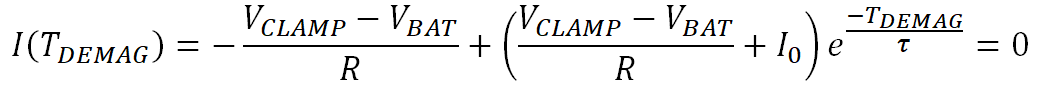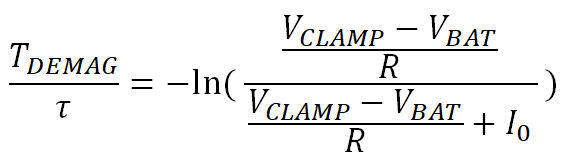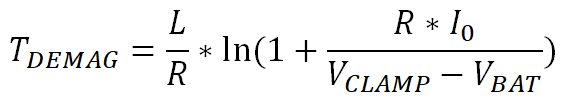SLVAE30E February   2021  – March 2021

2. 1Introduction
3. 2Driving Resistive Loads
1. 2.1 Background
2. 2.2 Application Example
3. 2.3 Why Use a Smart High Side Switch?
4. 2.4 Selecting the Right Smart High Side Switch
4. 3Driving Capacitive Loads
1. 3.1 Background
2. 3.2 Application Examples
3. 3.3 Why Use a Smart High Side Switch?
5. 4Driving Inductive Loads
6. 5Driving LED Loads
7. 6Appendix
8. 7References
9. 8Revision History

### 4.5.1 Demagnetization Time

The demagnetization time TDEMAG is the time that the current takes to decay from I0 to zero. This parameter is necessary to later calculate the total demagnetization energy.

To calculate TDEMAG, Equation 38 can be solved for when the total current is equal to 0.

Equation 39.Equation 40.Equation 41.Equation 42.Equation 42 shows that the demagnetization time is proportional to the time constant L/R and increases with larger I0, lower VCLAMP, and higher VBAT.